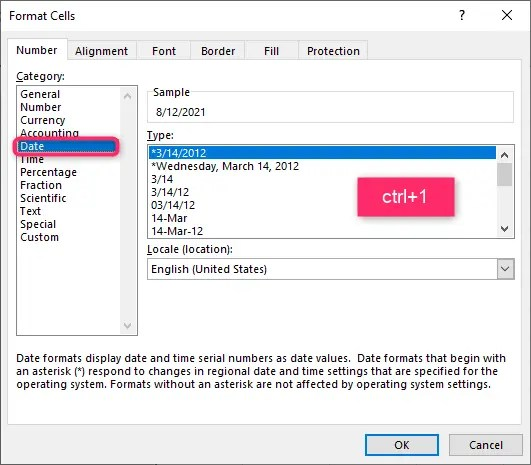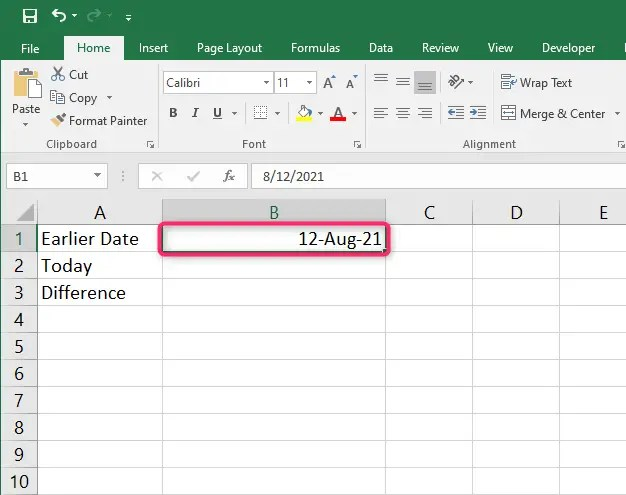# How to count days since date in Excel

Excel is a sophisticated program. It helps users manage and visualize data. However, working with days can be a complicated task.

You can easily count days since date in Excel that have passed since certain entries in your worksheet, and you can also count days separating dates in Excel.

Below, we give you a guide on calculating or counting the days since the date in Excel. Let’s get started.

## Steps to follow to count days since date in Excel

By subtracting the date from today, you can easily find out how many days have passed from a certain date. You can use the formula:

### Use TODAY() function and subtraction

For instance, let’s look days that have passed since your previous birthday. Here, you will have to calculate days by subtracting your birthday date from the current date.

Example:

Today is the 19th of July 2022 and your last birthday was on the 12th of August 2021. When calculating this in Excel using the Today() formula, you will find out 341 days have passed since your birthday.

Apart from this, the TODAY() formula can count the number of days between now and a later date.

In our example above, here are the steps to follow:

First, format the cells to date. Press ctrl+1 and navigate to the dateChoose your preferred date format and press okayEnter an early date on one cellUse =today() function to write the date today.  Alternatively, you can write the date manuallyOn a new cell. use subtraction to find the difference between today and the earlier## Use the DAYS function to count days since date in Excel

You can also use Excel’s DAYS function to return the of days separating two dates. Here you will use the formula:

=DAYS (end_date, start_date)Also, when you reverse the start date and end date, the DAYS function returns negative number as the answer.

For example, with your starting date in cell A2 and final date in cell A3, your formula will be:

=DAYS (A3, A2)

The formula will return your results as follows:

=A3 – A2

Apart from this, DAYS formula also handles dates in text format when Excel recognizes the text as a date.

For example;

=DAYS(“19-Jul-22″,”12-Aug-21”) // RETURNS 341 as the count of days since.### Using the DATEDIF function in Excel to calculate days till today

The DATEDIF function is used to calculate and count days between Excel dates. Here is the formula to use:

=DATEDIF (start_date, end_date, unit)

Unit is the time unit of years which can either be years, days or months.

1. In an open Excel worksheet, select a blank cell.

2. Type in the formula above referencing the cells with the specific data.

3. Press Enter and autofill any remaining cells.